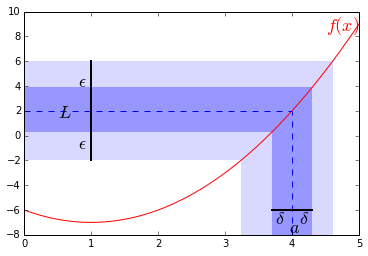# 极限

## 极限(Limits)

    f = lambda x: x**2-2*x-6
x = np.linspace(0,5,100)
y = f(x)

plt.plot(x,y,'red')
plt.grid('off')

l = plt.axhline(-8,0,1,linewidth = 2, color = 'black')
l = plt.axvline(0,0,1,linewidth = 2, color = 'black')

l = plt.axhline(y=2,xmin=0,xmax=0.8,linestyle="--")
l = plt.axvline(x=4,ymin=0,ymax=float(5)/9, linestyle = "--")

l = plt.axhline(-6,3.7/5,4.3/5,linewidth = 2, color = 'black')
l = plt.axvline(1,6.0/18,14.0/18,linewidth = 2, color = 'black')

p = plt.axhspan(-2,6,0,(1+sqrt(13))/5,alpha = 0.15, ec = 'none')
p = plt.axvspan((1+sqrt(5)),(1+sqrt(13)),0,1.0/3,alpha = 0.15, ec = 'none')

p = plt.axhspan(f(3.7),f(4.3),0,4.3/5,alpha = 0.3, ec = 'none')
p = plt.axvspan(3.7,4.3,0,(f(3.7)+8)/18,alpha = 0.3, ec = 'none')

plt.axis([0,5,-8,10])

plt.text(0.8,-1,r"$\epsilon$", fontsize = 18)
plt.text(0.8,4,r"$\epsilon$", fontsize = 18)
plt.text(3.75,-7.0,r"$\delta$", fontsize = 18)
plt.text(4.1,-7.0,r"$\delta$", fontsize = 18)
plt.text(3.95,-7.8,r"$a$", fontsize = 18)
plt.text(4.5,8.5,r"$f(x)$", fontsize = 18,color="red")

plt.show()Python中求该极限方法如下：

    x = sympy.Symbol('x',real = True)
y = f(x)
print y.limit(x, 2)
# result is: 2


## 泰勒级数用于极限计算## 洛必达法则（l'Hopital's rule）